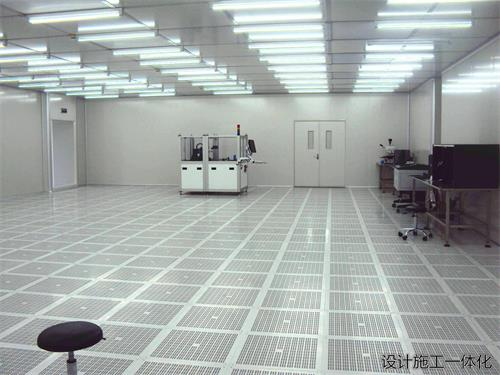# 制药车间洁净空调二次回风系统实例节能分析

摘要：药厂洁净空调特点是洁净区面积较大、洁净级别要求相对较高，在设计中，大多数项目采用一次回风系统，但使用一次回风系统，空调、制冷设备投资相应提高；二次回风系统设计不但能满足洁净区温湿度及洁净度要求，而且在运行中能节省能源的消耗。本文以某洁净车间为例，比较二次回风与一次回风的能耗情况。从计算结果可以得出结论，在正常运行过程中，二次回风系统比一次回风系统要更节能一些。

制药车间洁净空调系统为满足室内洁净度、温湿度、风压等要求，大多采用一次回风系统。一次回风系统设计、施工、调试都比较简单，相对而言，二次回风系统就要复杂的多。但在一次回风系统中，全部送风量都要经过空调机组的热湿处理，机组表冷器、加热器型号较大，设备初投资及运行费用较高；而二次回风系统中空调机组的表冷器、加热器只需处理系统的部分风量，也就可以选择较小的型号，降低了空调系统的能耗，同时也降低了设备初投资及运行费用。下面以笔者参与设计的某制药车间的一个洁净空调系统为例，对二次回风系统的节能效果进行分析。
1、设计基础数据
某水针车间配料、灌装区面积为360m2，洁净级别为C级。
洁净区室内设计参数：
温度tN=23±2℃，相对湿度φ=55±5%，焓值iN=48kJ/kg，含湿量dN=9.7g/kg
室外设计计算参数：
夏季室外计算干球温度tW=34.2℃，湿球温度ts=27.8℃，相对湿度φ=61.8%，焓值iW=89.2kJ/kg，含湿量dW=21.3g/kg
室内热湿负荷：
夏季冷负荷Q=80.46kW，散湿量W=0.0081kg/s；
为满足室内洁净度要求，系统送风量G=33700m3/h，系统新风比为32%。
2、一次回风系统能耗分析
热湿比ε=Q/W=80.46/0.0081=9933
根据送风量G及冷负荷Q确定送风焓差：
iN-iO=Q/G=80.46/33700/3600×1.2=7.16kJ/kg；
iO= iN-7.16=48-7.16=40.84 kJ/kg；
根据热湿比ε及室内状态点在焓湿图上确定送风状态点O：
dO=9.0g/kg，tO=18℃；
过O点在焓湿图上做dO=9.0g/kg的等绝对湿度线与φ=95%的等相对湿度线相交得空气处理过程的机器露点L：
tL=13.2℃，iL=36.1 kJ/kg
由系统新风比及室内外空气状态点参数，得室内外空气混合点C的焓值：
iC=（G1iN+ GWiW）/（ G1+ GW）
=（7.638×48+3.595×89.2）/（7.638+3.595）=61.18 kJ/kg
将以上各点在焓湿图上标注，系统空气处理流程如图1所示。
空气处理过程耗冷量为：
Q0=G（iC-iL）=11.233（61.18-36.1）=281.7kW
空气处理过程再热量为：
Q0=G（iO-iL）=11.233（40.84-36.1）=53.2kW
图1、一次回风系统空气处理流程
3、二次回风系统能耗分析
热湿比ε=Q/W=80.46/0.0081=9933
根据送风量G及冷负荷Q确定送风焓差：
iN-iO=Q/G=80.46/33700/3600×1.2=7.16kJ/kg；
iO= iN-7.16=48-7.16=40.84 kJ/kg；
根据热湿比ε及室内状态点在焓湿图上确定送风状态点O：
dO=9.0g/kg，tO=18℃；
在焓湿图上向下延长ε线与φ=95%的等相对湿度线相交得空气处理过程的机器露点L：
tL=11.6℃，iL=32.3kJ/kg；
根据G/GL=（ iN-iL）/（ iN-iO）得经过表冷器处理的空气量：
GL= G （ iN-iO）/（ iN-iL） =11.233×7.16/15.7=5.123kg/s
则二次回风量G2=G-GL=11.233-5.123=6.11kg/s
由新风比32%计算得新风量为10784m3/h（3.595kg/s），则一次回风量
G1= GL-GW=5.123-3.595=1.528kg/s
根据GW/GL=（ iC-iN）/（ iW-iN）得一次混合点C焓值：
iC=GW（ iW-iN）/ GL+ iN=3.595（89.2-48）/5.123+48=76.91kJ/kg
将以上各点在焓湿图上标注，系统空气处理流程如图2所示。
空气处理过程耗冷量为：
Q=GL（iC-iL）=5.123（76.91-32.3）=228.5kW
4、结论
经过以上计算可得，二次回风系统消耗冷量为一次制冷系统冷量的81%。
比一次回风系统节省的冷量为：
281.7-228.5=53.2kW
由于洁净空调系统对室内温湿度要求比较严格，在一次回风系统中夏季空气再热是必须的，而由于二次回风系统可充分利用室内余热，空气处理过程中也就不需再热，可节省再热量53.2kW。由此可以看出，洁净空调系统采用二次回风，不仅可以降低空调系统的初投资、运行费用，而且配套的制冷系统、制热系统的初投资及运行费用也可以降低。
x